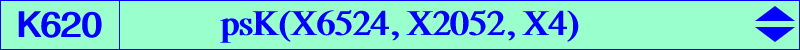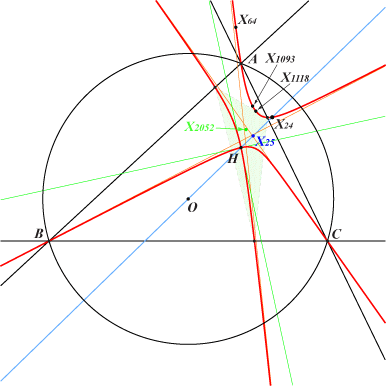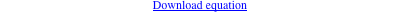too complicated to be written here. Click on the link to download a text file.X(4), X(24), X(64), X(1093), X(1118), X(2207), X(41320), X(43678), X(43742, X(52641), X(52646) cevian points of X(2052)K620 is an example of nodal cubic of Table 43. It is the cubic (K) obtained when P = X(24). K620 has two nodal tangents at H parallel to the asymptotes of the Jerabek hyperbola and three asymptotes parallel to those of the pivotal isogonal cubic whose pivot is the reflection X(11413) of X(24) about O. K620 is a psK with pseudo-pole X(6524) = H^3, pseudo-pivot X(2052), pseudo-isopivot X(25). See details here. K620 is the barycentric product H x K555. Its isogonal transform is K1324.